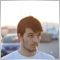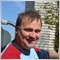# Grid lot increment fail1616

Lot increment is failing when Martingale < 1.5

```               double NextLong=OrderOpenPrice()-(GridSize*10)*Point;
double GridLots=NormalizeDouble(OrderLots()*Martingale,2);
RefreshRates();
return;
}```

Anyone knows how to fix that?22302

1. "Failing" like work is meaningless. "Doesn't work" is meaningless - just like saying the car doesn't work. Doesn't start, won't go in gear, no electrical, missing the key, flat tires - meaningless.

2. Use the debugger or print out your variables, including _LastError and prices and find out why.

3. `&&!OrderSend( …`
OrderSend does not return a boolean.1616

William Roeder:
1. "Failing" like work is meaningless. "Doesn't work" is meaningless - just like saying the car doesn't work. Doesn't start, won't go in gear, no electrical, missing the key, flat tires - meaningless.

2. Use the debugger or print out your variables, including _LastError and prices and find out why.

3. OrderSend does not return a boolean.

1. Code doesn't work because failing in two or three issues.

2. I think the function doesn't store decimal values below the rounding up point.

3. This OrderSend fit my needs, and the world will see when expert's working.1867

rounding means a values of 0.010000 to 0.0149999999 rounds down to 0.01 (for 2 digits)

rounding means a values of 0.015000 to 0.0199999999 rounds up to 0.02 (for 2 digits)

so if you use a martingale multiplier less then 1.5 and your previous lot size is 0.01 then your lot size will always be stay at 0.01.

example 0.01, then next lot size will be 0.01 and so on we go.

change your starting lot to 0.02 and you'll see that it works.

now the following is wrong

` double GridLots=NormalizeDouble(OrderLots()*Martingale,2);`

change this to something more intelligent so that it works starting at 0.01 also

double GridLots=NormalizeDouble(InitialLotSize * MathPow(Martingale, ExistingOpenOrderCount), 2)

you will need to calculate the value ExistingOpenOrderCount1616

Cornelis Duiker:

rounding means a values of 0.010000 to 0.0149999999 rounds down to 0.01 (for 2 digits)

rounding means a values of 0.015000 to 0.0199999999 rounds up to 0.02 (for 2 digits)

so if you use a martingale multiplier less then 1.5 and your previous lot size is 0.01 then your lot size will always be stay at 0.01.

example 0.01, then next lot size will be 0.01 and so on we go.

change your starting lot to 0.02 and you'll see that it works.

now the following is wrong

change this to something more intelligent so that it works starting at 0.01 also

double GridLots=NormalizeDouble(InitialLotSize * MathPow(Martingale, ExistingOpenOrderCount), 2)

you will need to calculate the value ExistingOpenOrderCount

Ok I got it, the MathPow formula is right.

But now it seems to be a problem in OrdersCount as I don't get the values exactly as in the excel sheet.

`GridLots=LotsOptimized()*MathPow(Martingale,SellOrders());`

When SellOrders() is:

```int SellOrders(){
int Short=0;
for(int i=0;i<OrdersTotal();i++){